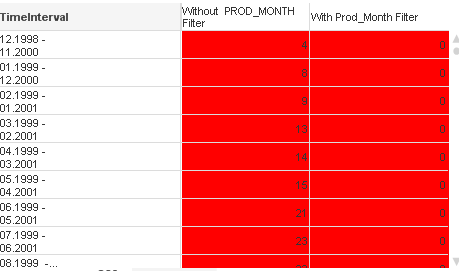# QlikView App Development

Discussion Board for collaboration related to QlikView App Development.

HighlightedPartner

## Why My Set Analysis not wokring

I have to sum up  field-A where Prod_Month is (> (Period_Month - V_Period_Length)<=Period_Month) and there are other criteria too ( Status = P , Age<=  V_Period_Length and Counter <= V_Period_Length).

i am using below expression:

sum({\$*<

[Status]={'P'},

[Counter]={'<\$(V_Period_Length)'},

[Age]={'<\$(V_Period_Length)'},

PROD_FISCAL_MONTH=,

PROD_FISCAL_YEAR=,

PROD_FISCYEAR=,

PROD_MONTH=,

PROD_DATUM=,

PROD_CALYEAR=,

PROD_CALQUARTER=,

PROD_CALMONTH=,

PERIOD_MONTH=,

PERIOD_MONTH_FORECAST=,

PERIOD_FISCAL_YEAR=,

PERIOD_FISCALMONTH=,

[Prod_Month] = {"<=\$(#=DATE(MAX(DATE(PERIOD_MONTH))))>\$(#=DATE(DATE#(MONTHEND(DATE(MAX(DATE(PERIOD_MONTH)),'DD.MM.YYYY'),-\$(V_Period_Length)),'DD.MM.YYYY')))"}

>} ANZRW)

I am using this expression in straight table in column named "With Prod_MOnth Filter" where Dimension is

=Date(addmonths(PERIOD_MONTH,-(V_Period_Length-1)), 'MM.YYYY') &' -'&chr(10)& Date(PERIOD_MONTH, 'MM.YYYY')

Problem is this expression is returning right result only if i select PRIOD_MONTH specifically otherwise wrong result. Kindly help here.Thanks,

Pragya